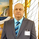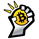533 просмотров
Noro's SILA v1.2 - these are 5 trend indicators in 1, for the sake of better accuracy.

1) Settings
2) Arrows

Noro's SILA v1.2 uses 5 trend indicators:

1) SuperTrend
2) DI Plus-Minus
3) WOW trend indicator (my idea)
4) BarColor indicator (my idea)
5) BestMA (or "BMA") indicator (my idea)

The user can switch-off any indicator from 5 to achieve big accuracy.

How does it work?

Each indicator from 5 defines a trend in own way. If two indicators report that there will be a uptrend, and three others the indicator report that there will be a downtrend - it is downtrend (a red background).

For an example

Now SuperTrend = uptrend = +1
Now DI Plus-Minus = downtrend = -1
Now WOW trend indicator = downtrend = -1
Now BarColor indicator = downtrend = -1
Now BestMA (or "BMA") indicator = uptrend = +1

Sum = + 1 - 1 - 1 - 1 + 1 = -1 = downtrend

If sum > 0 = uptrend

Sensivity

The user himself chooses what there will be a sensitivity (in settings).

If sensivity = 3:

sum > or = 3 - uptrend
sum < or = -3 - downtrend
sum > -3 and < 3 - NA-color of background

Trendlines

3 lower trendlines (blue plots) is "sum+3"
5 upper trendlines is "sum-5"
etc

Settings:

1) sensivity - you see above
2) distance - distance between the price and lines (for convenience)

## КомментарииWhat lines have you added or changed? Thanks.
Ответитьsyracusepro
@syracusepro, These are "SILA-lines" (my idea). Lines show strength of trend ("SILA" is russian word = "strength"). The more SILA-lines, the probability of change of a trend is less. Many lines under the price it is good for long entry. And vice versa. Many SILA-lines over the price it is good for the short entry. Between SILA-lines there is a distance. What will be a distance the user in settings chooses. If the price has crossed the SILA-line that nothing means. If a strength of trend sum = +3, then under the price is 3 SILA-lines. Price level of SILA-line = SMA(high, 5) + distance for upper lines. Or SMA(low, 5) - distance (for lower lines). SILA-line #2 is distance * 2, etc.
Ответитьnoro
@noro, code:

pricehi = sma(high, 5)
pricelo = sma(low, 5)
per = usetl == true ? dist / 1000 : 0
plot(pricelo * (1 - per), color=color1, linewidth=1, title="SILA-line")
Ответитьnoro
@noro, Very well done. I am in version 1.4. I also had to translate this for Thinkorswim, thinkscript and it is working fine. Thanks a lot.
Ответить